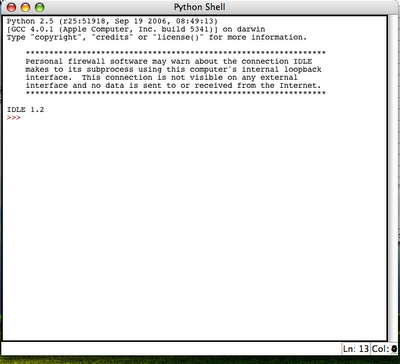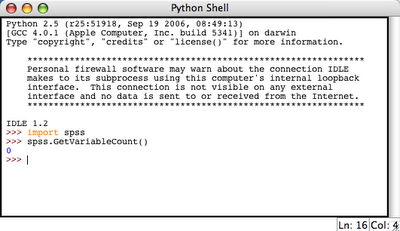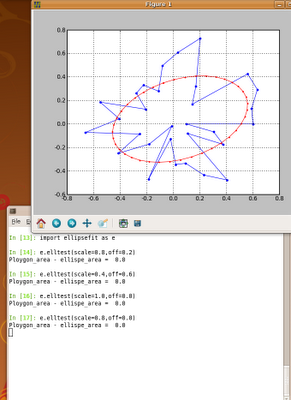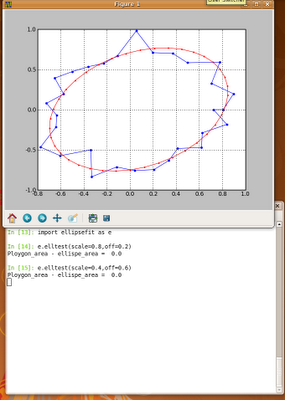## Sunday, December 21, 2008

### SPSS: programing SPSS with Python (part 1)

SPSS is a data mining and statistical analysis software. Resent versions (v14 - v17) allow to use Python to control SPSS. This can be quite handy, especially if there are lots of variables and lots of data files to analyze and such analysis is often repeated. In such a case, writing some script in Python that can execute SPSS analysis automatically can save you lots of time. This is the first post out of three, which will present some example of using Python and SPSS together. First, let me present what soft and hardware I have:
• Intel Mac X 10.4.11
• SPSS 16.0.1 with SPSS-Python Integration Plug-In
• Python 2.5

#### Check if SPSS-Python Integration Plug-In works

Before we can proceed any further we have to check if SPSS-Python Integration Plug-In is working, and we can control SPSS using Python. To do this I usually use the following procedure:
1. Start SPSS.
2. Create new script (File->New->Script):This results in a python IDLE console:3. Try to import spss library and execute some simple spss function (e.g. spss.GetVariableCount()):This resulted in 0 which is OK, because we do not have any active dataset.

So it appears that SPSS-Python union is working. Therefore, we can do something useful now.

#### Note

More information on SPSS-Python programming along with examples can be found in SPSS Programming and Data Management.

## Thursday, December 18, 2008

### Blogger: uploading files to blogger posts

By default you can upload only image or movie files into your blogger posts. To upload anything else, such as a zip file, music file it is necessary to have some host server that can store the files. Once the files are stored on a host, you can put a link to a file. So the question is where I can host my files - preferably for free. For me, the answer are Google sites. The reason is that it allows you to create five websites each containing up to 100 MBs of stuff. You can upload files there in a form of attachments or as so-called File Cabinet:Once the File Cabinet is created user can upload some files. And the links to these files can be posted in your blog post.### Example

As an example I have upload an zip file that contains SyntaxHighlighter 1.5.1 with simple index.html demo code snippets. The zip file is here.

## Wednesday, December 17, 2008

### Python: Ellipse fitting

The algorithm below fits an ellipse into the polygon (or set of points) in such a way that the area of the ellipse fitted is equal to the area of the polygon. `from __future__ import divisionfrom scipy import *import pylab as pdef ellipse(ra,rb,ang,x0,y0,Nb=50): '''ra - major axis length rb - minor axis length ang - angle x0,y0 - position of centre of ellipse Nb - No. of points that make an ellipse based on matlab code ellipse.m written by D.G. Long, Brigham Young University, based on the CIRCLES.m original written by Peter Blattner, Institute of Microtechnology, University of Neuchatel, Switzerland, blattner@imt.unine.ch ''' xpos,ypos=x0,y0 radm,radn=ra,rb an=ang co,si=cos(an),sin(an) the=linspace(0,2*pi,Nb) X=radm*cos(the)*co-si*radn*sin(the)+xpos Y=radm*cos(the)*si+co*radn*sin(the)+ypos return X,Y def _makexy(scale): x=reshape(array(range(scale*2)*(scale*2)),(scale*2,scale*2)) return x,x.T def _inside2(x,y,px,py): '''Check if point (x,y) inside polygon deifined by (px,py). From: http://www.ariel.com.au/a/python-point-int-poly.html ''' poly=zip(px,py) n = len(poly) inside =False p1x,p1y = poly for i in range(n+1): p2x,p2y = poly[i % n] if y > min(p1y,p2y): if y <= max(p1y,p2y): if x <= max(p1x,p2x): if p1y != p2y: xinters = (y-p1y)*(p2x-p1x)/(p2y-p1y)+p1x if p1x == p2x or x <= xinters: inside = not inside p1x,p1y = p2x,p2y return inside def _polyMask(X,Y,rs): '''Make binary mask of polygon. Values of one in 2D array pd represents points enclosed by the polygon.''' pd=zeros((rs*2,rs*2)) for i in range(pd.shape): for j in range(pd.shape): if _inside2(i,j,X,Y)==True: pd[i,j]=1.0 return pddef ellfit(X,Y,rs=50,showFig=True): '''Fit and ellipse to a polygon defined by X,Y in such a way that area of the ellispe fitted is the same as area of the polygon. Based on ellfit.pro by D.G. Long, Brigham Young University. Conditions: 1. The polygon must not be self intersecting. 2. Polygon must be closed (last point must match the first one). 3. Polygon must be centered in (0,0) 4. Polygon must be within rectangle <-1,1>x<-1,1> ''' #Xmax,Ymax=abs(X).max(),abs(Y).max() #X=X/Xmax #Y=Y/Ymax pi2=2*pi rs2=2*rs if showFig: fig = p.figure(figsize=(5,5)) ax = fig.add_subplot(111) X=X*rs+rs Y=Y*rs+rs xa,ya=_makexy(rs) pd=_polyMask(X,Y,rs) if showFig: p.plot(X,Y,"rs-",ms=15) for i in range(pd.shape): for j in range(pd.shape): if pd[i,j]==1.0: pass p.plot([i],[j],'g.',ms=10) m00=pd.sum() m10=(xa*pd).sum() m01=(ya*pd).sum() xm,ym=m10/m00, m01/m00 u20=(xa*xa*pd).sum()-xm*m10 u02=(ya*ya*pd).sum()-ym*m01 u11=(xa*ya*pd).sum()-ym*m10 tn2=2*u11/(u20-u02) ang=arctan(tn2)/2.0 if u02>u20: ang=ang-pi/2 xmean,ymean=X.mean(),Y.mean() Xr = cos(ang) * (X-xmean) - sin(ang) * (Y-ymean) +xmean Yr = sin(ang) * (X-xmean) + cos(ang) * (Y-ymean) +ymean t=_polyMask(Xr,Yr,rs) m00=t.sum() m10=(xa*t).sum() m01=(ya*t).sum() xm,ym=m10/m00, m01/m00 u20=(xa*xa*t).sum()-xm*m10 u02=(ya*ya*t).sum()-ym*m01 u11=(xa*ya*t).sum()-ym*m10 aa=((16*u20**3)/(pi2*u02))**(1/8) bb=((16*u02**3)/(pi2*u20))**(1/8) ##ellispe params a,b=max([aa,bb]),min([aa,bb]) b=t.sum()/(pi*a) ecc=sqrt(a**2-b**2) theta=ang ellArea=pi*a*b print "Ploygon_area - ellispe_area = ",round(ellArea-t.sum(),3) #print time.time()-t1 if showFig: p.grid(True) p.show() #xee,yee=b/rs*cos(0),a/rs*sin(0) #b3,a3=xee*max([Xmax,Ymax])/cos(theta),yee*min([Xmax,Ymax])/sin(theta) #print b3/a3, b/a return a/rs,b/rs,ecc,thetadef elltest(scale=0.8,off=0.2): #generate an example, random, non-self-intersecting polygon. #This is done by first generating #it in polar coordinates and than translating it #to cartesian. Theta1,R1=linspace(0,2*pi,30),rand(30)*scale+off X1,Y1=R1*cos(Theta1),R1*sin(Theta1) X1=append(X1,X1) Y1=append(Y1,Y1) p.plot(X1,Y1,".-",ms=10) a2,b2,ecc2,alpha2=ellfit(X1,Y1,showFig=False) Xe,Ye=ellipse(b2,a2,-alpha2,X1.mean(),Y1.mean(),Nb=40) p.plot(Xe,Ye,"r.-") p.grid(True) p.show() passif __name__ == '__main__': elltest()`

### Examples### Note 1

The algorithm has the following limitations:
• The polygon must not be self intersecting.
• Polygon must be closed (last point must match the first one).
• Polygon must be centered in (0,0)
• Polygon must be within rectangle <-1,1>x<-1,1>

### Note 2

This algorithm could be modified to overcome the above (Note 1) limitations. However, at the very moment I don't have time to do this. Hope this is not a problem.

### Note 3

Searching the Internet for an ellipse fitting script for Python I found fitellipse.py. It is very useful, however, sometimes I got an Exception that its not possible to fit an ellipse. The algorithm presented here seems to work good. In case the ellipse cannot be fitted try to increase rs attribute of ellfit function. The default value is 50 (rs=50).

### Note 3

This script is in ellipsefit.py.

## Sunday, December 14, 2008

### Some free, essential e-books

I have just found some free e-books source on www.techotopia.com. There are books such as:
• JavaScript essentials
• Ruby essentials
• PHP essentials
• MySQL essentials
• Xen essentials
• and more.

## Tuesday, December 09, 2008

### SciTE: customize it

SciTE is very nice little text editor. I constantly use it, but it's default layout settings are not good for me. However, it allows to create, so called User Option File (/home/me/.SciTEUser.properties) and adjust it to user's preferences. This is what I have in this file:`split.vertical=0line.margin.visible=1line.margin.width=4position.width=650position.height=920output.vertical.size=175`With these settings the editor looks as follows:## Wednesday, December 03, 2008

### ubuntu: mount windows share

I had ubuntu 8.10.
First I had to install samba and smbfs. Than I created mount point /home/me/ub1 and used the commad`sudo mount -t cifs -o username=myusername,password=mysecretpass,rw,uid=1000,gid=1000 //iptoshare/sharename /home/me/ub1`

## Tuesday, December 02, 2008

### Python: use Cython to make it faster

Just as an example, I present some function that I translated to Cython. I also show how big the change in speed of my application was. The function is a part of an image processing application and it analyzes a given image on pixel by pixel basis. There are four nested loops! in this function. For this reason the function is very time consuming. The original code was:`def calculateVar(self):`
` N=self.N;I=self.ima[ans,xoffs,yoffs,dists]=self.getSearchRegion()noOfAngles=int(ans.shape)self.ROTs=zeros(xoffs.shape)diffMtx_size=N*N/self._jump/self._jumpdiffMtx=zeros(diffMtx_size,dtype=int)for ai in range(noOfAngles): for offi in range(xoffs.shape+0): diffMtx.fill(0) ind=0; xoff=xoffs[ai,offi] yoff=-yoffs[ai,offi] for y1 in range(0,I.shape,self._jump): for x1 in range(0,I.shape,self._jump): x2=x1+xoff y2=y1+yoff if x2>=N or y2>=N or x2<0 ind="ind+1" diffmtx2="diffMtx[diffMtx">0] self.ROTs[ai,offi]=diffMtx2.var() `
This function was change to the following one:`def calculateVar(self):N=self.N;I=self.imaI2=array(I,dtype=int)[ans,xoffs,yoffs,dists]=self.getSearchRegion()#this is part in Cython!self.ROTs=loopcore.loopcore(I2,ans.shape,xoffs.shape, array(xoffs,dtype=int),array(yoffs,dtype=int), N, self._jump)`
where loopcore is a Cython module loopcore.pyx as follows:`import numpy as npcimport numpy as npDTYPE = np.intctypedef np.int_t DTYPE_tctypedef np.float_t DTYPE_t2cdef inline int int_abs(int a, int b): return abs(a-b)def loopcore(np.ndarray[DTYPE_t, ndim=2] I,int noOfAngles,int noOfpixels, np.ndarray[DTYPE_t, ndim=2] xoffs, np.ndarray[DTYPE_t, ndim=2] yoffs,int N, int jump ):cdef int y1,x1,x2, y2, ind,ai,offi, xoff,yoff,array_sizearray_size=N*N/jump/jumpcdef np.ndarray[DTYPE_t, ndim=1] p= np.zeros(array_size, dtype=DTYPE)cdef np.ndarray[DTYPE_t, ndim=1] p2= np.zeros(0, dtype=DTYPE)cdef np.ndarray[DTYPE_t2, ndim=2] ROTs= np.zeros([noOfAngles,noOfpixels], dtype=np.float)for ai in range(noOfAngles):for offi in range(noOfpixels):p.fill(0)ind=0xoff=xoffs[ai,offi]yoff=-yoffs[ai,offi]for y1 in range(0,N,jump): for x1 in range(0,N,jump): x2=x1+xoff y2=y1+yoff if x2>=N or y2>=N or x2<0 ind="ind+1" p2="p[p">0]ROTs[ai,offi]=p2.var()return ROTs`
The gain in speed was huge. Execution of this function in Python takes about 2.10 min, while using Cython it takes about 0.05 min, i.e. code in Cython is 40+ times faster than that in Python. I'm sure that it can be made even faster than that!

Note 1
I noticed that the more variables is defined (cdef, int, float, ...) the greater the gain in speed is achieved.

Note 2
In Ubuntu 8.04 and 8.10 I compiled the Cython pyx files without any problems using the following command:` cython loopcore.pyx`` gcc -shared -pthread -fPIC -fwrapv -O2 -Wall -fno-strict-aliasing -I/usr/include/python2.5 -o loopcore.so loopcore.c`
Note 3
When running any python code from this post, remember to correct code indentations.